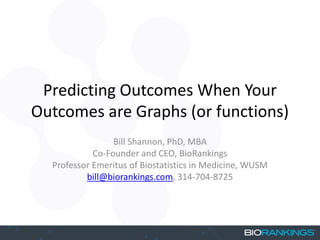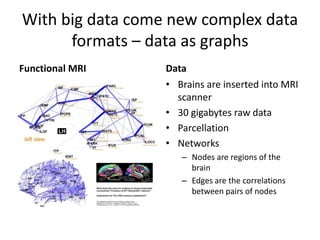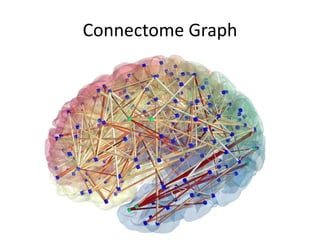Successfully reported this slideshow.

# Predicting Outcomes When Your Outcomes are Graphs - StampedeCon AI Summit 2017×

# Predicting Outcomes When Your Outcomes are Graphs - StampedeCon AI Summit 2017

In many modern applications data are collected in unusual form. Connectome or brain imaging data are graphs. Wearable devices measuring activity are functions over time. In many cases these objects are collected for each individual or transaction leaving the statistician with the challenge of analyzing populations of data not in classical numeric and categorical formats in big spreadsheets. In this talk I introduce object oriented data analysis with an application we recently developed for regression analysis. This talk will be aimed at the general data scientist and emphasis on the concepts and not mathematical detail. The take home message is how can we use covariates (i.e., meta-data) to predict what the structure of a brain image graph will be.

In many modern applications data are collected in unusual form. Connectome or brain imaging data are graphs. Wearable devices measuring activity are functions over time. In many cases these objects are collected for each individual or transaction leaving the statistician with the challenge of analyzing populations of data not in classical numeric and categorical formats in big spreadsheets. In this talk I introduce object oriented data analysis with an application we recently developed for regression analysis. This talk will be aimed at the general data scientist and emphasis on the concepts and not mathematical detail. The take home message is how can we use covariates (i.e., meta-data) to predict what the structure of a brain image graph will be.

### Predicting Outcomes When Your Outcomes are Graphs - StampedeCon AI Summit 2017

1. 1. Predicting Outcomes When Your Outcomes are Graphs (or functions) Bill Shannon, PhD, MBA Co-Founder and CEO, BioRankings Professor Emeritus of Biostatistics in Medicine, WUSM bill@biorankings.com, 314-704-8725
2. 2. With big data come new complex data formats – data as graphs Functional MRI Data • Brains are inserted into MRI scanner • 30 gigabytes raw data • Parcellation • Networks – Nodes are regions of the brain – Edges are the correlations between pairs of nodes
3. 3. Connectome Graph
4. 4. With big data come new complex data formats – data as graphs Data Microbiome • Sample from human, animal, field (soil), environment • Next Generation Sequencing (write once, read never data) • Genomic analysis processing – Annotation to taxonomic label (i.e., genus, species)
5. 5. Microbiome Tree
6. 6. Statistics is interested in inferring things about everything from a sample Sample to Population Inference • Collect a bunch of graphs – 1 per subject • Plot graphs • Estimate mean and variance (or g* and tau) • Does this plot teach us about the graphs in terms of how they are distributed and what the central tendency is?
7. 7. Does this plot teach us anything?
8. 8. Graphs are too complex – let’s simplify Network metrics Average connectivity Small world network Species diversity Taxa counts Enterotype
9. 9. Many-to-one mapping is not necessarily a good way to simplify data for analysis
10. 10. Simplifying in fMRI and Microbiome fMRI • Average Node Connectivity • Consider two brain scans – Patient 1 • Right half ANC = 10 • Left half ANC = 0 – Patient 2 • Right half ANC = 5 • Left half ANC = 5 • Both whole brain ANC = 5 Microbiome • Species Diversity • Consider two samples – Patient 1 • Proportion Taxa A, B, C = 1/3 • Proportion Taxa D, E, F = 0 – Patient 2 • Proportion Taxa A, B, C = 0 • Proportion Taxa D, E, F = 1/3 • Both have Simpson diversity = 0.33
11. 11. We analyze graphical data the same way as we analyze columns of data Gibbs distribution • Let G be a finite set of graphs and denote the elements of G by g. Let 𝑑 be an arbitrary distance metric on G. The Gibbs distribution on the graphs G is denoted by ℙ 𝒈; 𝒈∗ , 𝝉 = 𝒄 𝒈∗ , 𝝉 𝒆𝒙𝒑 −𝝉𝒅 𝒈∗ , 𝒈 , ∀𝒈 𝝐 𝐆, with parameters g∗ the central or average graph, and 𝜏 a non-negative number that is a measure of the dispersion of the observed connectome data around g∗ . 𝑐 g∗ , 𝜏 is the normalizing constant. ℙ 𝑔𝑖; g∗ , 𝜏 is the probability of observing a specific graph 𝑔𝑖 given the parameters g∗ , 𝜏 . Statistics on Graphs
12. 12. We analyze graphical data the same way as we analyze columns of data Recursive partitioning • Regress the graphs on covariates • In this example of Parkinson's disease – Y = connectome – X = group, sex, age • RP splits the connectomes into homogeneous groups based on likelihood of Gibbs Statistics on Graphs
13. 13. What else can be analyzed with graphical OODA? IoT Blockchain Cybersecurity
14. 14. What about data which are functional objects? Untargeted Metabolomics • Liquid chromatography and mass spec – LC/MS • RT x m/z plots • Which peaks correspond to metabolites (known or unknown), and which peaks are different in patients who live and die?
15. 15. RT x m/z plots are too complex – let’s simply Looking for things that look different and then testing them statistically is wrong – P values don’t mean anything in these cases.
16. 16. Why not analyze functions using functional OODA?
17. 17. Why not analyze functions using functional OODA?
18. 18. Field Enabling Technology Bioinformatics Exploratory Analysis Translational Statistics Microbiome Next generation Sequencing Assembly, annotation, chimera checking Cluster analysis, multidimensional scaling, heatmaps Dirichlet- multinomial for taxa counts Gibbs distribution for taxonomic trees Brain Imaging Functional MRI (fMRI) Image registration, parcellation Generalized linear models with multiple testing adjustment, graph metrics Gibbs distribution for connectome Metabolomics LC/MS Peak detection, centering Mass univariate testing with multiple testing adjustment Functional data analysis, Gibbs distribution, Co- Inertia, and the Exploratory- Validation Model for experimental design Projects in object oriented data analysis